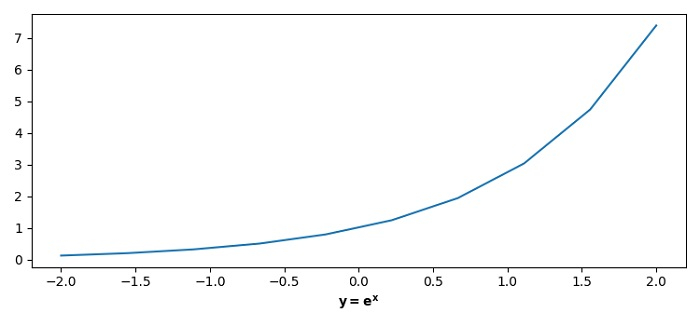# Increasing the space for X-axis labels in Matplotlib

To increase the space for X-axis labels in Matplotlib, we can use the spacing variable in subplots_adjust() method's argument.

## Steps

• Set the figure size and adjust the padding between and around the subplots.

• Create a new figure or activate an existing figure using figure() method.

• Create x and y data points using numpy.

• Plot x and y using plot() method.

• Put xlabel using xlabel() method with LaTex expression.

• Use subplots_adjust() method to increase or decrease the space for X-axis labels

• To display the figure, use show() method.

## Example

import numpy as np
from matplotlib import pyplot as plt
plt.rcParams["figure.figsize"] = [7.50, 3.50]
plt.rcParams["figure.autolayout"] = True
fig = plt.figure()
x = np.linspace(-2, 2, 10)
y = np.exp(x)
plt.plot(x, y)
plt.xlabel("$\bf{y=e^{x}}$")
spacing = 0.100
plt.show()